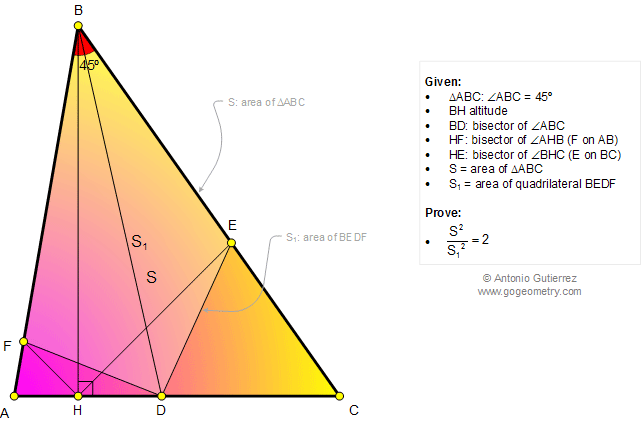< PREVIOUS PROBLEM  |  NEXT PROBLEM >

Math Geometry Problem 1016. Triangle, 45 Degrees, Area, Quadrilateral, Altitude, Angle Bisector

 The figure below shows a triangle ABC of area S with angle A = 45 degrees, BH altitude, and BD angle bisector. HF is the bisector of angle AHB (F on AB) and HE is the bisector of angle BHC. If S1 is the area of quadrilateral BEDF, prove that S2 / S12 = 2.## Mind Map: George Pólya's 1945 book, "How to Solve It"

George Pólya's 1945 book "How to Solve It, A new aspect of Mathematical Method", is a book describing methods of problem solving. It suggests the following steps when solving a mathematical problem: (1) First, you have to understand the problem. (2) After understanding, then make a plan. (3) Carry out the plan. (4) Look back on your work. How could it be better?
A mind map is a diagram used to visually organize information, knowledge, concepts or ideas.Home | SearchGeometry | Problems | All Problems | Open Problems | Visual Index | 10 Problems | Problems Art GalleryArt | 1011-1020 | Triangle | Angle Bisector | 45 degrees | Altitude | Quadrilaterals | Areas | Email | Solution / comment | by Antonio Gutierrez
Last updated: Sep 19, 2014